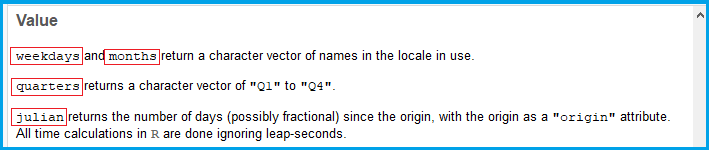# weekdays, months, quarters & julian Functions in R (4 Examples)

In this article, I’ll explain how to apply the R programming functions weekdays, months, quarters, and julian. Let’s have a look at the basic R syntax and the definition of the four functions:

Basic R Syntax of weekdays, months, quarters & julian:

```weekdays(date)
months(date)
quarters(date)
julian(date, origin = date_origin)```

Definition of weekdays, months, quarters & julian:

The weekdays, months, and quarters functions return the corresponding weekday, month, or quarter of a date object as character string.

The julian function returns the number of days between two date objects as character string.

This tutorial will show you based on four examples how to use the weekdays, months, quarters, and julian functions in R.

Let’s dive into it!

## Example 1: weekdays Function in R

In the first example I’ll show you how to find the weekday of a date object in R. Before we can start with the example, we need to create such a date object for the following examples:

```date <- as.POSIXlt("2020-05-10")          # Create example date
date                                      # Print date to RStudio console
# "2020-05-10 CEST"```

As you can see, our date object contains the date 2020-05-10 CEST.

To this date object, we can now apply the weekdays R function in order to find the corresponding day of the week:

```weekdays(date)                            # Apply weekdays function
# "Sunday"```

The weekdays function returns Sunday to the RStudio console. In other words: The 10th of May in the year 2020 is a Sunday.

## Example 2: months Function in R

Similar as before in Example 1, we can apply the months function to extract the month of our date object:

```months(date)                              # Apply months function
# "May"```

The date 2020-05-10 CEST corresponds to the month May (not shocking, I know). Even though the month of a date object might be obvious, the months command can be very useful for a vector containing many date objects.

## Example 3: quarters Function in R

Example 3 shows how to find the quarter of a date object in R. Have a look at the following R code:

```quarters(date)                            # Apply quarters function
# "Q2"```

Our example date is in the second quarter (i.e. Q2) of the year.

## Example 4: julian Function in R

The julian function can be used to compute the number of days between two dates. Let’s create a second date object for the next example:

`date_origin <- as.POSIXlt("1950-05-10")   # Create second date object`

Now we can apply the julian R function to calculate the number of days between our two date objects:

```julian(date, origin = date_origin)        # Apply julian function
# Time difference of 25567.96 days```

The time difference between the 10th of May 1950 and the 10th of May 2020 is 25567.96 days.

## Tutorial Video & Further Resources for the Handling of Dates in R

I have also published a video tutorial on this topic, so if you are still struggling with the code, watch the following video on my YouTube channel:

Dealing with date objects in R can be quite difficult. For that reason, I have listed some further resources for the handling of dates below. First of all, you might have a look at the R help documentation of the weekdays Function. There you will find further examples and definitions for the weekdays, months, quarters, julian functions. Especially helpful are the following few lines of the help documentation, which define the four functions in further detail:Figure 1: R Help Documentation of weekdays, months, quarters & julian.

Furthermore, you could have a look at the following video of the YouTube channel thenewboston. In the video, the speaker explains how to work with R date objects in general:

Last but not least, you also may have a look at the other R programming tutorials in this website. I have published a few articles on the handling of dates (and many other topics) already:

From this tutorial, you should have learned how to apply weekdays, months, quarters, and julian. However, if you have any further questions, let me know in the comments below.

Subscribe to the Statistics Globe Newsletter